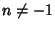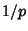## Artin's Constant

Ifandis not a Perfect Square, then Artin conjectured that the Setof all Primes for whichis a Primitive Root is infinite. Under the assumption of the Extended Riemann Hypothesis, Artin's conjecture was solved in 1967 by C. Hooley. If, in addition,is not anth Power for any, then Artin conjectured that the density ofrelative to the Primes is(independent of the choice of), whereand the Product is over Primes. The significance of this constant is more easily seen by describing it as the fraction of Primesfor whichhas a maximal Decimal Expansion (Conway and Guy 1996).

References

Conway, J. H. and Guy, R. K. The Book of Numbers. New York: Springer-Verlag, p. 169, 1996.

Finch, S. Favorite Mathematical Constants.'' http://www.mathsoft.com/asolve/constant/artin/artin.html

Hooley, C. On Artin's Conjecture.'' J. reine angew. Math. 225, 209-220, 1967.

Ireland, K. and Rosen, M. A Classical Introduction to Modern Number Theory, 2nd ed. New York: Springer-Verlag, 1990.

Ribenboim, P. The Book of Prime Number Records. New York: Springer-Verlag, 1989.

Shanks, D. Solved and Unsolved Problems in Number Theory, 4th ed. New York: Chelsea, pp. 80-83, 1993.

Wrench, J. W. Evaluation of Artin's Constant and the Twin Prime Constant.'' Math. Comput. 15, 396-398, 1961.